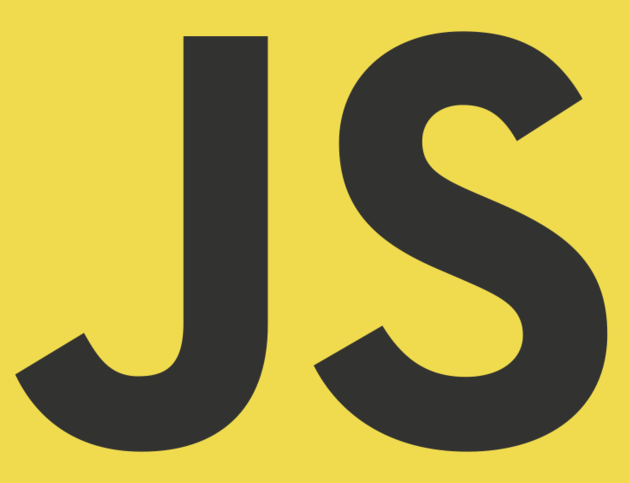# How to convert the Number String to Number Array?

Recently, when I was working on a requirement, got a scenario to convert the number string like the below to Number Array.

``let stringOfNumbers = "1,2,3";``

Used the below code to convert that Number string to Number Array.

``let numberArray = stringOfNumbers.map(x => Number(x));``

Click on the link to see the code.

Hope this code helped you to convert the Number string to Number Array.Arun PottiAuthor: Arun Potti